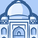# New to Qlik Sense

If you’re new to Qlik Sense, start with this Discussion Board and get up-to-speed quickly.

Announcements
cancel
Showing results for
Did you mean:Contributor

## Comparative Analysis Syntax

Hi,

I created a comparative analysis dashboard with alternate states that compares two different time periods. I would like to create a measure that gives me the delta sales for a specific region. This is the syntax I have so far, but the error I get is "')' expected."

(sum(({\$*[Time Period 1]} geography1={'NA'}) invoiced_amount1)-sum(({\$*[Time Period 2]} geography1={'NA'}) invoiced_amount1))/fabs(sum(({\$*[Time Period 2]} geography1={'NA'}) invoiced_amount1))

My equation is essentially: (Time Period 1 Sales-Time Period 2 Sales)/Time Period 2 Sales

My alternate states are "Time Period 1" and "Time Period 2". The sales field is "invoiced_amount1". The region field is "geography1" and I want to analyze the data where geography1=NA.

I'm not sure where my error is. Please assist, thank you!

Labels (4)

• ### SaaS

1 Solution

Accepted SolutionsMVP

May be this

``````(Sum({\$*[Time Period 1]<geography1 = {'NA'}>} invoiced_amount1) -
Sum({\$*[Time Period 2]<geography1 = {'NA'}>} invoiced_amount1))
/
fabs(Sum({\$*[Time Period 2]< geography1 = {'NA'}>} invoiced_amount1))``````
2 RepliesMVP

May be this

``````(Sum({\$*[Time Period 1]<geography1 = {'NA'}>} invoiced_amount1) -
Sum({\$*[Time Period 2]<geography1 = {'NA'}>} invoiced_amount1))
/
fabs(Sum({\$*[Time Period 2]< geography1 = {'NA'}>} invoiced_amount1))``````Contributor
Author

Worked perfectly. Thank you!